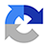# Albright / FoxMathematical Modeling with Excel

2. New Auflage 2019, 360 Seiten, Gebunden, Format (B × H): 406 mm x 194 mm, Gewicht: 856 g Reihe: Textbooks in Mathematics
ISBN: 978-1-138-59707-5
Verlag: Taylor & Francis Ltd

## Albright / FoxMathematical Modeling with Excel

This text presents a wide variety of common types of models found in other mathematical modeling texts, as well as some new types. However, the models are presented in a very unique format. A typical section begins with a general description of the scenario being modeled. The model is then built using the appropriate mathematical tools. Then it is implemented and analyzed in Excel via step-by-step instructions. In the exercises, we ask students to modify or refine the existing model, analyze it further, or adapt it to similar scenarios.

## Weitere Infos & Material

Chapter 1 -- What is Mathematical Modeling? Chapter 2 -- Proportionality and Geometric Similarity Chapter 3 -- Empirical Modeling Chapter 4 -- Discrete Dynamical Systems Chapter 5 -- Differential Equations Chapter 6 -- Simulations Chapter 7 -- Optimization

Albright, Brian (Concordia University)
Brian Albright grew up in Colby, KS; received undergraduate degrees in mathematics and secondary education from Emporia State University, Emporia, KS; and received a D.Sc. in systems science and mathematics from Washington University in St. Louis. In early 2004 he worked as a civilian transportation analyst at the United States Transportation Command. Since 2004 he has taught all levels of undergraduate mathematics at Concordia University, Seward, NE. He has published two textbooks, Mathematical Modeling with Exceland Essentials of Mathematical Statistics; has written two articles for The College Mathematics Journal; and has written ancillary material for several textbooks.

## Ihre Fragen, Wünsche oder Anmerkungen

Ihre Nachricht*
Wie möchten Sie kontaktiert werden?
Anrede*
Titel
Vorname*
Nachname*